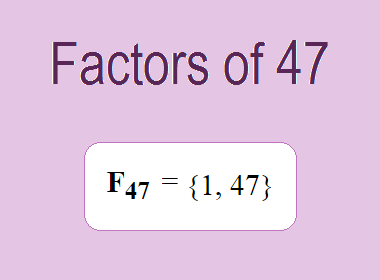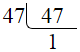# Factors of 47The factors of 47 are 1 and 47 i.e. F47 = {1, 47}. The factors of 47 are all the numbers that can divide 47 without leaving a remainder. 47 is a prime number, so it is divisible by 1 and 47 only.

We can check if these numbers are factors of 47 by dividing 47 by each of them. If the result is a whole number, then the number is a factor of 47. Let's do this for each of the numbers listed above:

·        1 is a factor of 47 because 47 divided by 1 is 47.

·        47 is a factor of 47 because 47 divided by 47 is 1.

## Properties of the Factors of 47

The factors of 47 have some interesting properties. One of the properties is that the sum of the factors of 47 is equal to 48. We can see this by adding all the factors of 47 together:

1 + 47 = 48

Another property of the factors of 47 is that the only prime factor of 47 is 47 itself.

********************

********************

## Applications of the Factors of 47

The factors of 47 have several applications in mathematics. One of the applications is in finding the highest common factor (HCF) of two or more numbers. The HCF is the largest factor that two or more numbers have in common. For example, to find the HCF of 47 and 94, we need to find the factors of both numbers and identify the largest factor they have in common. The factors of 47 are 1, and 47. The factors of 94 are 1, 2, 47, and 94. The largest factor that they have in common is 47. Therefore, the HCF of 47 and 94 is 47.

Another application of the factors of 47 is in prime factorization. Prime factorization is the process of expressing a number as the product of its prime factors. The prime factor of 47 is 47 since it is only the prime number that can divide 47 without leaving a remainder. Therefore, we can express 47 as:

47 = 47

We can do prime factorization by division method as given below,47 = 47

Since 47 is a prime number, there is no factor tree of 47.

## Conclusion

The factors of 47 are the numbers that can divide 47 without leaving a remainder. The factors of 47 are 1, and 47. The factors of 47 have some interesting properties, such as having a sum of 48. The factors of 47 have several applications in mathematics, such as finding the highest common factor and prime factorization.